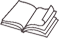# Reasoning with Data

## An Introduction to Traditional and Bayesian Statistics Using R

HardcoverPaperbacke-bookprint + e-book
Hardcover
May 19, 2017
ISBN 9781462530274
Price: \$95.00 \$71.25
325 Pages
Size: 7" x 10"
Paperback
May 22, 2017
ISBN 9781462530267
Price: \$45.00 \$33.75
325 Pages
Size: 7" x 10"
e-book
April 28, 2017
PDF ?
Price: \$45.00 \$33.75
325 Pages
print + e-book
Paperback + e-Book (PDF) ?
Price: \$90.00 \$49.50
325 PagesProfessors: free copies available for adoption consideration

Introduction

Getting Started

1. Statistical Vocabulary

Descriptive Statistics

Measures of Central Tendency

Measures of Dispersion

Distributions and Their Shapes

Conclusion

Exercises

2. Reasoning with Probability

Outcome Tables

Contingency Tables

Conclusion

Exercises

3. Probabilities in the Long Run

Sampling

Repetitious Sampling with R

Using Sampling Distributions and Quantiles to Think about Probabilities

Conclusion

Exercises

4. Introducing the Logic of Inference Using Confidence Intervals

Exploring the Variability of Sample Means with Repetitious Sampling

Our First Inferential Test: The Confidence Interval

Conclusion

Exercises

5. Bayesian and Traditional Hypothesis Testing

The Null Hypothesis Significance Test

Replication and the NHST

Conclusion

Exercises

6. Comparing Groups and Analyzing Experiments

Frequentist Approach to ANOVA

Bayesian Approach to ANOVA

Finding an Effect

Conclusion

Exercises

7. Associations between Variables

Null Hypothesis Testing on the Correlation

Bayesian Tests on the Correlation Coefficient

Categorical Associations

Exploring the Chi-Square Distribution with a Simulation

The Chi-Square Test with Real Data

Bayesian Approach to Chi-Square Test

Conclusion

Exercises

8. Linear Multiple Regression

Bayesian Approach to Linear Regression

A Linear Regression Model with Real Data

Conclusion

Exercises

9. Interactions in ANOVA and Regression

Interactions in ANOVA

Interactions in Multiple Regression

Bayesian Analysis of Regression Interactions

Conclusion

Exercises

10. Logistic Regression

A Logistic Regression Model with Real Data

Bayesian Estimation of Logistic Regression

Conclusion

Exercises

11. Analyzing Change over Time

Repeated Measures Analysis

Time-Series Analysis

Exploring a Time Series with Real Data

Finding Change Points in Time Series

Probabilities in Change-Point Analysis

Conclusion

Exercises

12. Dealing with Too Many Variables

Internal Consistency Reliability

Rotation

Conclusion

Exercises

13. All Together Now

The Big Picture

Appendix A. Getting Started with R

Running R and Typing Commands

Installing Packages

Quitting, Saving, and Restoring

Conclusion

Appendix B. Working with Data Sets in R

Data Frames in R

Reading Data Frames from External Files

Appendix C. Using dplyr with Data Frames

References

Index